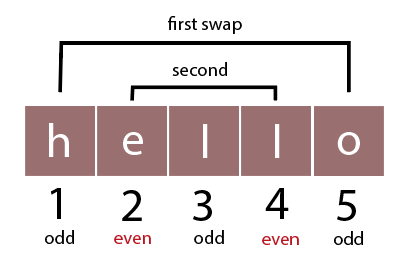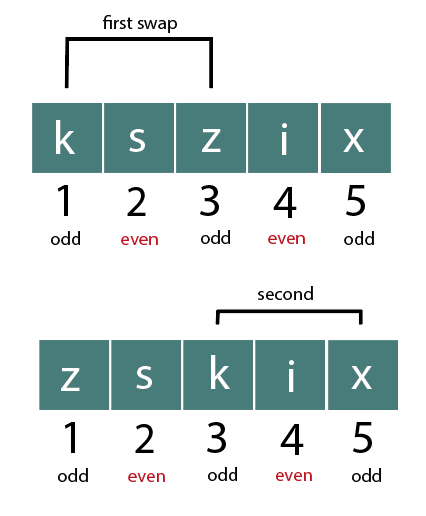# Lexicographically Largest String using at most K Swaps at Same Parity Indices

## Introduction

Lexicographic order is the generic order in which alphabets and symbols are sequenced. Starting from A to Z, Z is the largest alphabet, while A is the smallest.  Considering we have a string ‘str’ and a positive integer ‘k’, our task will be to find the lexicographically largest string possible in str using k swaps at the same parity indices. At the same parity, indices signify that the elements to be swapped must be at the even or odd indices.

Let us now have a look at some examples for better understanding:

### Sample Examples

First, the trick is to assess all the elements at odd and even indices, respectively, decide which ones are the largest lexicographically, and then plan swaps accordingly.

Example 1:

Input:

``str=hello, k=2``

Output:

``olleh``

Considering 1-indexing,

Largest to smallest elements at odd indices: o, l, h

Largest to smallest elements at even indices: l, eIn the first swap, we will interchange ‘e’, and ‘l’ at even indices, and the string will become ‘hlleo’.

In the second swap, we will interchange ‘o’ and ‘h’ at odd indices. The string will now be ‘olleh’

Example 2:

Input:

``kszix, k=2``

Output:

``zsxik``

Largest to smallest elements at odd indices: z, x, k

Largest to smallest elements at even indices: s, iIn the first swap, we will interchange ‘k’, and ‘z’ at odd indices, and the string will become ‘zskix’.

In the second swap, we will interchange ‘k’ and ‘x’ at even indices. The string will now be ‘zsxik’

(Also see Data Structures)

## Brute force approach

The most straightforward approach is to make the leftmost elements the maximum possible. The largest element on odd indices can first be brought to index 1 first. The largest element on even indices can be brought to index 2. The second largest element on odd indices can be brought to index 3 and so on. Then, we will repeat the procedure K times.

### Implementation in C++

``````#include<bits/stdc++.h>
using namespace std;
void findMax(string s , int k)
{
int n = s.length();
int count = 0;
int max, index = 0;
for (int i = 0; i < n; i++)
{
max = s[i];
for (int j = i + 2; j < n; j += 2)
{
if (s[j] > max)
{
index = j;
max = s[j];
}
}
if (index != 0)
{
swap(s[i], s[index]);
index = 0;
count++;
}
if (count == k)
break;
}
cout << s << endl;
}
int main()
{
string s = "salty";
int k = 2;
findMax(s, k);
}``````

Output:

``ytlas``

### Implementation in Java

``````import java.util.*;

public class Main {
static String swap(String str, int i, int j)
{
StringBuilder sb = new StringBuilder(str);
sb.setCharAt(i, str.charAt(j));
sb.setCharAt(j, str.charAt(i));
return sb.toString();
}

public static void findMax(String s , int k)
{
int n = s.length();
int count = 0;
int max, index = 0;
for (int i = 0; i < n; i++)
{
max = s.charAt(i);
for (int j = i + 2; j < n; j += 2)
{
if (s.charAt(j) > max)
{
index = j;
max = s.charAt(j);
}
}
if (index != 0)
{
s=swap(s,i, index);
index = 0;
count++;
}
if (count == k)
break;
}
System.out.println(s);
}

public static void main(String args[]) {
String s = "salty";
int k = 2;
findMax(s, k);
}

}``````

Output:

``ytlas``

### Implementation in Python

``````​​def swap(str,i,j):
str=list(str)
temp1=str[i]
str[i]=str[j]
str[j]=temp1
string1=""
return string1.join(str)

def findMax(s , k):
n = len(s)
count = 0
maxi=0
index = 0
for i in range(0,n):
maxi = s[i]
j=i+2
while(j<n):
if (s[j] > maxi):
index = j
maxi = s[j]
j+=2

if (index != 0):
s=swap(s,i, index)
index = 0
count+=1

if (count == k):
break
print(s)

s = "salty";
k = 2;
findMax(s, k);``````

Output:

``Ytlas``

### Complexity Analysis

Time Complexity: O(N^2)

Since the brute force approach uses nested for loops to compare elements at the same parity indices, the time complexity of such an approach will be O(N^2).

Note that lexicographically largest string problems are all different and have different conditions, such as having a limited number of swaps, only allowing some particular swaps(in this case only even or only odd indices), etc. However, the basic approach toward such problems will remain the same. We could use techniques like bubble sort for lesser constraints and simpler problems, just swapping according to the condition for the brute force, or using priority queues for the least time complexity.

Space complexity: O(1)

The space complexity will be O(1) as we are not using any extra space and have just used variables for maintaining the answer.

## Efficient approach

The efficient approach uses two priority queues (one for even indices and one for odd) for more convenience.

### Steps of Algorithm

1. Create two priority queues for even and odd indices, respectively.
2. Use a loop to iterate elements at even indices. If an element is larger than the previous element on the left, swap them in the priority queue holding the elements.
3. Repeat the procedure for odd elements.
4. The loop has to run ‘k’ a number of times. Therefore, we will terminate the loop when k becomes 0.

### Implementation in C++

``````#include <bits/stdc++.h>
using namespace std;

string largestLexicographic(string str, int k)
{
int n = str.length();
priority_queue<pair<char, int> > OddPQ, EvenPQ;

// storing every possible even pair
for (int i = 2; i < n; i = i + 2)
{

// storing the pair with character and index
EvenPQ.push(make_pair(str[i], i));
}

// storing every possible odd pair
for (int i = 3; i < n; i = i + 2)
{

// storing the pair with character and index
OddPQ.push(make_pair(str[i], i));
}

for (int i = 0; i < n; i++)
{

// even indices
if (i % 2 == 0)
{

// removing unrequired pairs
while (!EvenPQ.empty()
and EvenPQ.top().second <= i)
EvenPQ.pop();

// character greater than current character
if (!EvenPQ.empty()
and EvenPQ.top().first > str[i])
{

swap(str[i], str[EvenPQ.top().second]);

int idx = EvenPQ.top().second;
EvenPQ.pop();
EvenPQ.push({ str[idx], idx });
k--;
}
}
// odd indices
else
{

// removing unrequired pairs
while (!OddPQ.empty()
and OddPQ.top().second <= i)
OddPQ.pop();

// character greater than current character
if (!OddPQ.empty()
and OddPQ.top().first > str[i])
{

swap(str[i], str[OddPQ.top().second]);
int idx = OddPQ.top().second;
OddPQ.pop();
OddPQ.push({ str[idx], idx });
k--;
}
}
if (k == 0)
break;
}
return str;
}

// calling function
int main()
{
string str = "salty";
int k = 2;

cout << largestLexicographic(str, k);
return 0;
}``````

Output:

``ytlas``

### Implementation in Java

``````import java.util.*;
class Pair {
public char key;
public int value;
public Pair(char key, int value) //Constructor of the class
{
this.key = key;
this.value = value;
}
public void print(){
System.out.println("< "+key+", "+value+ " >");
}

public char getKey(){
return this.key;
}

public int getValue(){
return this.value;
}
}

class cmp implements Comparator<Pair> {
public int compare(Pair p1, Pair p2)
{
if(p1.key < p2.key){
return 1;
}
else{
if(p1.key > p2.key){
return -1;
}
}
return 0;
}
}

public class Main {
static String swap(String str, int i, int j)
{
StringBuilder sb = new StringBuilder(str);
sb.setCharAt(i, str.charAt(j));
sb.setCharAt(j, str.charAt(i));
return sb.toString();
}

static String largestLexicographic(String str, int k)
{
int n = str.length();
PriorityQueue<Pair> EvenPQ=
new PriorityQueue<Pair>(new cmp());
PriorityQueue<Pair> OddPQ=
new PriorityQueue<Pair>(new cmp());
// PriorityQueue<Pair<Integer,Integer> > pq=
// new PriorityQueue<Pair<Integer,Integer>>(n, Comparator.comparing(Pair::getKey));

// storing every possible even pair
for (int i = 2; i < n; i = i + 2)
{

// storing the pair with character and index
// EvenPQ.peek().print();
}

// storing every possible odd pair
for (int i = 3; i < n; i = i + 2)
{

// storing the pair with character and index
// OddPQ.peek().print();
}

for (int i = 0; i < n; i++)
{

// even indices
if (i % 2 == 0)
{

// removing unrequired pairs
while (!EvenPQ.isEmpty() && EvenPQ.peek().value <= i){
EvenPQ.poll();
}

// character greater than current character
if (!EvenPQ.isEmpty() && EvenPQ.peek().key > str.charAt(i))
{

str=swap(str,i,EvenPQ.peek().value);

int idx = EvenPQ.peek().value;
EvenPQ.poll();
k--;
}
}
// odd indices
else
{

// removing unrequired pairs
while (!OddPQ.isEmpty() && OddPQ.peek().value <= i )
{
OddPQ.poll();

}

// character greater than current character
if (!OddPQ.isEmpty() && OddPQ.peek().key > str.charAt(i))
{

str=swap(str,i, OddPQ.peek().value);
int idx = OddPQ.peek().value;
OddPQ.poll();
k--;
}
}
if (k == 0)
break;
}
return str;
}

public static void main(String args[]) {
String str = "salty";
int k = 2;
System.out.println(largestLexicographic(str, k));
return ;
}

}
``````

Output:

``ytlas``

### Implementation in Python

``````def swap(str,i,j):
str=list(str)
temp1=str[i]
str[i]=str[j]
str[j]=temp1
string1=""
return string1.join(str)

def largestLexicographic(str,k):
n = len(str);
EvenPQ = []
OddPQ = []

# storing every possible even pair
i=2
while(i<n):

# storing the pair with character and index
EvenPQ.append([str[i], i])
EvenPQ.sort(reverse = True)
# print(EvenPQ," ",EvenPQ,"\n")
i=i+2

# storing every possible odd pair
i=3
while(i<n):

# storing the pair with character and index
OddPQ.append([str[i], i])
OddPQ.sort(reverse = True)
# print(OddPQ," ",OddPQ,"\n")
i=i+2

for i in range(0,n):

# even indices
if (i % 2 == 0):

# removing unrequired pairs
while (len(EvenPQ)!=0 and EvenPQ <= i):
EvenPQ.pop()

# character greater than current character
if (len(EvenPQ)!=0 and EvenPQ > str[i]):

str=swap(str,i, EvenPQ)

idx = EvenPQ
EvenPQ.pop()
EvenPQ.append([ str[idx], idx ])
EvenPQ.sort(reverse=True)
k-=1

# odd indices
else:

# removing unrequired pairs
while (len(OddPQ)!=0 and OddPQ <= i):
OddPQ.pop()

# character greater than current character
if (len(OddPQ)!=0 and OddPQ > str[i]):

str=swap(str,i, OddPQ)
idx = OddPQ
OddPQ.pop()
OddPQ.append([ str[idx], idx ])
OddPQ.sort(reverse=True)
k-=1

if (k == 0):
break
return str

# calling function
str = "salty";
k = 2;

print(largestLexicographic(str, k));

``````

Output:

``ytlas``

### Complexity Analysis

Time complexity: O(N log N)

The running time will grow in proportion to the log of input size, i.e., the size of the string and then multiplied by the number of times swaps take place (depending on the value of ‘k’). Therefore the time complexity for the efficient approach to finding the lexicographically largest string will be O(N log N).

Space complexity: O(N)

For every input element, there is a fixed number of bytes allocated. Therefore the space complexity is O(N).

### What is the lexicographical order in Java?

The words are sorted in lexical, lexicographic, or dictionary order in Java-or any other language for the matter-means that they are alphabetically ordered based on their component alphabets.

### What alphabet is the smallest in lexicographic order?

'A' has the smallest lexicographic order, which keeps going up until 'Z', the largest lexicographic. Therefore the smallest possible string for the first 5 alphabets lexicographically is “abcde”.

### How to decide if a swap should be made in lexicographic decisions?

While making lexicographical decisions, a swap must be made if the alternative is better than the current element.

## Conclusion

In this article, we learned about lexicographic strings. We studied two approaches to solve lexicographic problems and discussed the time and space complexity of the code provided.